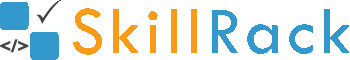C Program - Find if a given number (integer) is a Prime number or not1 year ago

The below C program checks and then prints if a given number is Prime or not without using any in-built functions like square root.

Instead of square root we use divisor*divisor <= N so that the value of divisor goes up only till square root. Please note that checking divisibility only till square root is optimal than checking for divisibility till N/2.

#include <stdio.h>

int isPrime(int N){
if(N <= 1){
return 0;
}

for(int divisor=2; divisor*divisor <= N; divisor++){
if(N%divisor == 0){
return 0;
}
}
return 1;
}

int main()
{
int N;
scanf("%d",&N);
printf(isPrime(N) ? "Prime":"Not Prime");
return 0;
}